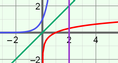# Logarithmic Functions

### DESCRIPTION

Compare the equation of a logarithmic function to its graph. Change the base of the logarithmic function and examine how the graph changes in response. Use the line y = x to compare the associated exponential function.

Full Lesson Info